﻿
Reach Us+44-7482-875032
Peristaltic Flow of a Sisko Fluid over a Convectively Heated Surface with Viscous Dissipation

# Journal of Applied & Computational MathematicsOpen Access

All submissions of the EM system will be redirected to Online Manuscript Submission System. Authors are requested to submit articles directly to Online Manuscript Submission System of respective journal.

# Peristaltic Flow of a Sisko Fluid over a Convectively Heated Surface with Viscous Dissipation

Shaheen A1* and Asjad MI2
1Department of Mathematics, Comsat Institute of Science and Technology, Park Road, Tarlai Kalan, Islamabad, Pakistan
2Department of Mathematics, University of Management and Technology, Block C-II, Johar Town, Lahore, Pakistan
*Corresponding Author: Shaheen A, Department of Mathematics, Comsat Institute Of Science And Technology, Park Road, Tarlai Kalan, Islamabad 45550, Pakistan, Tel: +92 51 9247000, Email: [email protected]

Received Date: Dec 26, 2017 / Accepted Date: Jan 03, 2018 / Published Date: Feb 16, 2018

Keywords: Sisko fluid; Peristaltic flow; Viscous-dissipation effects; Convective boundary condition; Exact solution

#### Introduction

waves on the walls of the channel which mix and transport fluid in a channel is termed as peristalsis. In several procedures of physiology and engineering, peristaltic flow concerns are broadly encountered in channel or tube. The applications of peristalsis covers swallowing food through the esophagus, urine transportation from kidney to bladder, assessment of chyme in gastrointestinal tract, ovum movement in the female fallopian tube, vasomotion of narrow blood vessels, movement of spermatozoa in human reproductive tract and water movement from ground to above branches of grown-up trees [1-4].

Peristaltic flows have many biological and industrial applications such as blood pumps in heart, lung machines and transportation of mordant fluids. For the viscous fluids, Lithium and Sharpio presented the earliest theoretical and experimental models for peristaltic transport [5,6]. Peristalsis during male reproductive system was examined experimentally and numerically by Srivastava, Gupta , Guha et al.  and Batra , where a peristaltic flow has been modelled in the vas deferens by considering it to be a non-uniform tube. Many modern mechanical procedures have been investigated on the primary of the peristaltic pumping for transporting fluids without internal moving parts, for example, the blood pump in the heart, lung machine and the peristaltic transport of noxious fluid in the nuclear industry. It was also clarified that in the case of hyperthermia, the tissue can be destroyed when heated upto 42°C−45°C. A mathematical model of peristaltic hydromagnetic flow in a tube for the Johnson-Segalman fluid has been studied by Hayat and Ali . Hydromagnetic flow of fluid in a uniform pipe with variable thickness was investigated by Hakeem et al. . Nadeem and Akbar  have studied the peristaltic wave of a non-Newtonian fluid in a non-uniform inclined pipe. Peristaltic transportation of a non-Newtonian fluid in an inclined channel was discussed by Vajravelu et al. .

Study of peristaltic flow in the presence of magnetic field has also achieved a lot of importance in daily life and engineering sciences. Some previous papers dealing with MHD flows of peristaltic are discussed [14-16]. Effects of MHD on the peristaltic flows for different modes of heat transfer like conduction, convection and radiations are reported [17-21]. For other studies regarding MHD flows, are can consult [22-24]. The rate of heat transfer is dependent on the temperatures of the systems and the properties of the prevailing medium through which the heat is transferred. Different authors have discussed [25,26] the effect of force on the heat convection and mass transfer. Study of peristaltic flow in the presence of magnetic field has also achieved a lot of importance in daily life and engineering sciences. Some previous papers dealing with MHD flows of peristaltic are discussed [14-16]. Effects of MHD on the peristaltic flows for different modes of heat transfer like conduction, convection and radiations [17-21]. For other studies regarding MHD flows [22-24].

Since the first investigation done by the Choi , the study of nanofluids have attracted the attention of many researchers due to its tremendous applications in various fields of life such as biomedical devices, treatment of tumor, nuclear reactor, microchips, cooling, radiators and nanomedicines etc. Only few researches are available on the peristaltic flows of nanofluids [28-33]. Motivated by the above work the aim of present findings is to treatise the Sisko fluid and viscous dissipation effects with convective boundary conditions. The problem of the two-dimensional motion of non-Newtonian fluid inside a uniform horizontal with convective boundary condition is discussed. The governing equations for conservation of mass, momentum and heat equation have been simplified throughout the assumption of low Reynolds number and long wave length approximation. The modelled equations are solved analytically by homotopy perturbation method . The physical features of pertinent parameters are discussed through graphs.

This paper is structured as follows: In this paper, we discuss the details of the mathematical formulation. This describes the proposed solution methodologies for the solution of the governing partial differential equations. This presented the analytical results and discussions. Finally, in the paper is concluded with a discussion on the results.

#### Mathematical Formulation

We have analyzed the peristaltic flow of an incompressible Sisko fluid in a uniform tube. The flow in the tube is sinusoidal wave along the wall with constant speed c. The wall of the tube are defined as,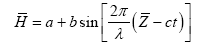(1)

The geometry of the problem is shown in Figure 1 where a is the radius of the tube at inlet.

b is the wave amplitude, λ represent the wavelength, c is the propagation speed and t is the time.

The fundamental equations of continuity, momentum, nanoparticle concentration are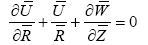(2)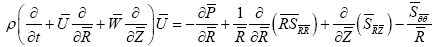(3)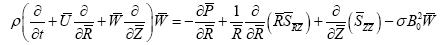(4)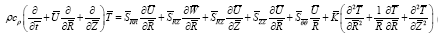(5)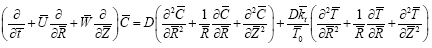(6)

The transformation relation between the two Coordinate frames are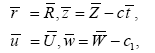The corresponding boundary conditions are defined as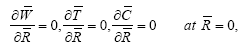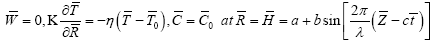The constitutive equation for a Sisko fluid model is defined as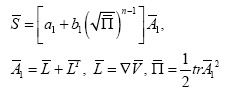The non-dimensional constants are known as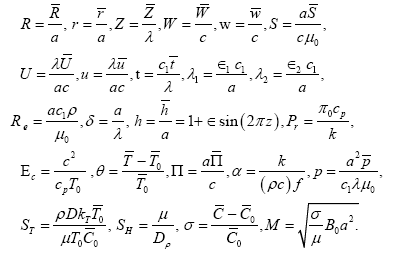We suppose for small Reynolds number Re << 1 and by the Longwavelength approximation δ << 1 the flow inside the passage is very slow. Therefore, neglecting the non-inertial terms we get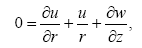(7)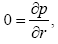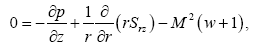(9)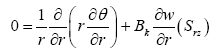(10)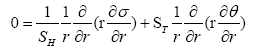(11)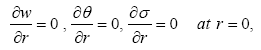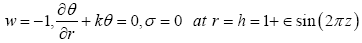where M is the Hartmann numbe r, b represent the sisko parameter and ∈ the amplitude ratio (∈=a/b), Soret number is ST, Schmidt number is the SH and Brinkman number is

Bk

where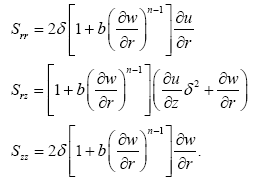Finally, in simplified form above equation can be written as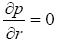(12)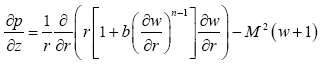(13)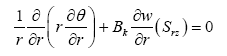(14)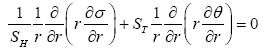(15)

The corresponding boundary conditions are defined as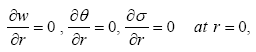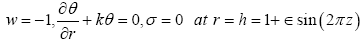#### Solution Methodology

Since the resulting equation of the above boundary value problem is non-linear. Thus it is appropriate homotopy perturbation method to solve eqn.(16), The homotopy perturbation method for eqn. (16) is defined as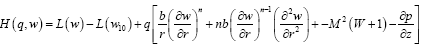(16)

We choose linear operator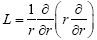and initial guess which satisfy the required boundary conditions are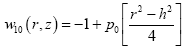(17)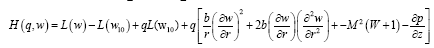(18)

where initial guess is w10 and embedding constant is q ∈ [0;1]. According to HPM, we solve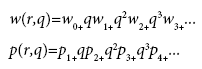The velocity field for q→1 takes the form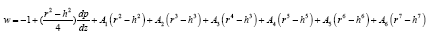(19)

The exact solutions for the temperature and concentration satisfying the relative boundary conditions are directly written as: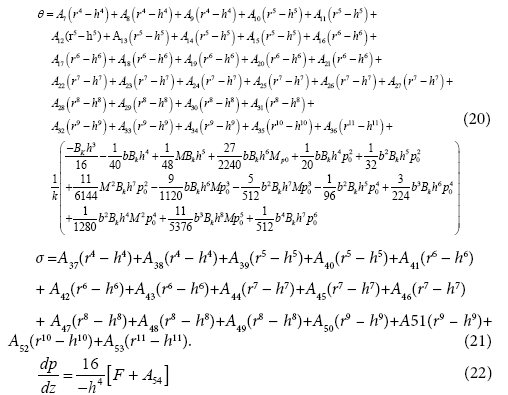Using Mathematica the constants A1, A2,..., A54 can be calculated from eqn. (22).

Flow rate in the dimensionless form can be written as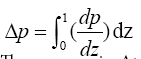(23)

The pressure rise Δp can be written as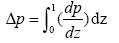Velocities in terms of stream functions are defined as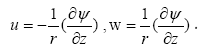For the flow analysis, we have considered three waveforms, namely, sinusoidal wave, trapezoidal wave and mulltisinusoidal wave. The dimensionless equations can be written as

1. Sinusoidal wave

h(z)=1+∈sin(2πz)

2. Multisinusoidal wave

h(z)=1+∈sin(2mπz)

3. Trapizoidal wave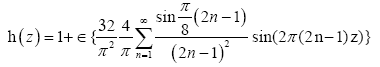4. Square wave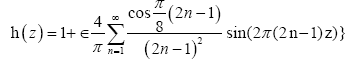#### Results and Discussions

In this section, we have analyzed the solution for physiological breakdown of Sisko fluid model with convective boundary condition in a uniform tube through graphs. We have presented the solution attained by HPM by framing velocity, pressure rise, pressure gradient, temperature, concentration and streamline graphs for diverse values of the parameters n (power law index) and b (fluid parameter) and M (magnetic hydrodynamics), κ (Biot number), Bk (Brinkman number), ST (Soret number), SH (Schmidt number) respectively. Figures 2-5 show that with the increase in b, Bk, M,κ temperature profile increases. From figure 5 it is seen that with the increase in κ temperature profile decreases. Figures 6-9 show that with the increase in b,Bk,M,ST concentration profile increases. From Figure 10 it is seen that with the increase in SH concentration profile decreases. Figure 11 shows that increases the value of M while the velocity profile in the centre of the tube decreases as well as it gets opposite behaviour nearest of the tube or near the peristaltic wave. Figures 12 and 13 show that shear stress gets increasing function in the region (0.5 ≤ r ≤ 0) whereas it get opposite behaviour in the region (−1 ≤ r ≤−0.49). Figure 14-19 show the pressure rise (versus flow rate) for diverse value of M,b,. From Figure 14, it is depicted that by increasing value of M pressure rise increasing in the region (Q ∈ [−2,0.5]) whereas reflux occur in the last. The retrograde pumping region can also be seen in Figure 14 when Q < 0 and Δp > 0 and free pumping region can be seen when Q = 0 and Δp = 0. Moreover, augmented pumping region can also be seen in Figure 14 when Q > 0 and Δp < 0. From Figures 16 and 18 it is seen that with the increase in pressure rise decreases in the region (Q ∈ [−2,−0.4]) whereas reflux occur in the last. Figures 15, 17 and 19 show the friction force for diverse values of M,b,. From these figures it is depicted that the friction forces have an opposite behavior as compared to pressure rise.

Figure 18: Effect of b on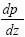Figure 19: Effect of M on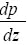Figures 20-22 describe the behavior of pressure gradient for different waveforms like Sinusoidal, multisinusoidal, trapezoidal. Figure 20 describe that increasing value of the pressure gradient decreases in the region (0 ≤ Z ≤ 0.5) and increases in the region (0.6 ≤ Z ≤ 1) and reflux occur in the region (1.1 ≤ Z ≤ 1.5).

Figure 20: Effect of ε on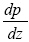Figures 21-23 show that the behavior for different values of by considering the multisinusoidal wave, trapezoidal. Figures 24 and 25, it is depicted that by increasing value of b,M pressure rise increasing. Figures 26-29 illustrate the streamlines for different wave shapes. It is distinguish that the streamlines of the flow are affected in a related behavior by increasing the value of ∈. In fact it is pragmatic that the strength of trapped bolus appear in the wider part of the tube decreases by increasing the value of ∈.

#### Conclusion

We have analyzed the peristaltic flow of non-Newtonian Sisko fluid with convective boundary conditions in a uniform tube. The viscous dissipation effects are also taken into account.

The main outcome of the present study is concisely as follows:

• Exact solution is premeditated for concentration field and temperature profile,

• The temperature profile is enhanced corresponding to increasing values of parameters b,M,Bk and decreases by increasing the values of κ,

• The concentration field is increases by increasing the values of b,M,Bk and decreases by increasing the values of SH,

• The pressure gradient increases with the increasing value of b,M,

There is an opposite behavior between pressure rise and the frictional forces.

#### References

Citation: Shaheen A, Asjad MI (2018) Peristaltic Flow of a Sisko Fluid over a Convectively Heated Surface with Viscous Dissipation. J Appl Computat Math 7: 402. DOI: 10.4172/2168-9679.1000402

Copyright: © 2018 Shaheen A, et al. This is an open-access article distributed under the terms of the Creative Commons Attribution License, which permits unrestricted use, distribution, and reproduction in any medium, provided the original author and source are credited.

Select your language of interest to view the total content in your interested language

##### Recommended Journals
Viewmore
###### Article Usage
• Total views: 746
• [From(publication date): 0-2018 - Aug 18, 2019]
• Breakdown by view type
• HTML page views: 700
• PDF downloads: 46

## Post your commentCan't read the image? click here to refresh
###### Peer Reviewed Journals

Make the best use of Scientific Research and information from our 700 + peer reviewed, Open Access Journals

International Conferences 2019-20

Meet Inspiring Speakers and Experts at our 3000+ Global Annual Meetings

Top